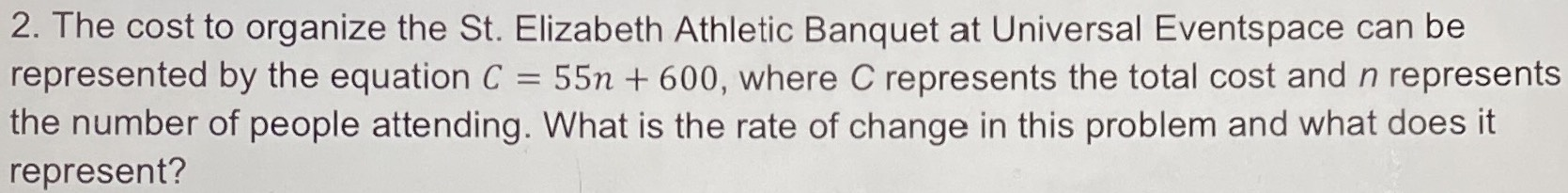### ¿Todavía tienes preguntas de matemáticas?

Pregunte a nuestros tutores expertos
Algebra
Pregunta2. The cost to organize the St. Elizabeth Athletic Banquet at Universal Eventspace can be represented by the equation $$C = 55 n + 600$$ , where $$C$$ represents the total cost and $$n$$ represents the number of people attending. What is the rate of change in this problem and what does it represent?